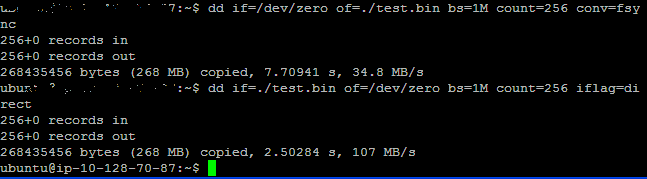# 正确使用 dd 测试磁盘读写速度

2013/08/04 01:56

dd bs=1M count=128 if=/dev/zero of=test

dd bs=1M count=128 if=/dev/zero of=test; sync

dd bs=1M count=128 if=/dev/zero of=test conv=fdatasync

dd bs=1M count=128 if=/dev/zero of=test oflag=dsync

答：区别在于内存中写缓存的处理方式。

dd bs=1M count=128 if=/dev/zero of=test

没有加任何参数，dd默认的方式不包括“同步(sync)”命令。也就是说，dd命令完成前并没有让系统真正把文件写到磁盘上。所以以上命令只是单纯地把这128MB的数据读到内存缓冲当中(写缓存[write cache])。所以你得到的将是一个超级快的速度。因为其实dd给你的只是读取速度，直到dd完成后系统才开始真正往磁盘上写数据，但这个速度你是看不到了。所以如果这个速度很快，先不要偷着乐。呵呵

dd bs=1M count=128 if=/dev/zero of=test; sync

和前面1中的完全一样。分号隔开的只是先后两个独立的命令。当sync命令准备开始往磁盘上真正写入数据的时候，前面dd命令已经把错误的“写入速度”值显示在屏幕上了。所以你还是得不到真正的写入速度。

dd bs=1M count=128 if=/dev/zero of=test conv=fdatasync

加入这个参数后，dd命令执行到最后会真正执行一次“同步(sync)”操作，所以这时候你得到的是读取这128M数据到内存并写入到磁盘上所需的时间，这样算出来的时间才是比较符合实际的。

dd bs=1M count=128 if=/dev/zero of=test oflag=dsync

加入这个参数后，dd在执行时每次都会进行同步写入操作。也就是说，这条命令每次读取1M后就要先把这1M写入磁盘，然后再读取下面这1M，一共重复128次。这可能是最慢的一种方式了，因为基本上没有用到写缓存(write cache)。

问：那应该用哪一种呢?

答：建议使用

dd bs=1M count=128 if=/dev/zero of=test conv=fdatasync

因为这种方式最接近计算机实际操作，所以测出来的数据最有参考价值。

PS：

# 用sync不靠谱, 最好还是在开机的时候把内存设的很小, 测试文件的大小远远大于内存才比较合理.0
18 收藏

### 作者的其它热门文章0 评论
18 收藏
0